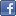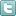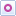EE Times-India > Sensors/MEMS

Sensors/MEMS# Utilising FETS for sensor applications (Part 2)

Posted: 10 Jul 2015Print Version

Keywords:op amps  FET  photodiode preamp  transresistance amplifiers  hydrophone

In figure 11, the effective feedback resistor is about 2MΩ because R6 and R7 provide a divide by two voltage divider. The effective feedback resistance is [1 + (R6/R7) ] x RF = [1 +1] x 1MΩ = 2MΩ, given that R1 << RF or 10KΩ << 1MΩ.

J1A and J1B are low noise and low capacitance matched pair JFETs such as the LSK489.

U1 can be typically a bipolar input stage op amp for the lowest equivalent input voltage noise density such as the AD797. Variable trimmer capacitor C3 is adjusted for the flattest frequency response.

Alternatively C3 may be adjusted for the best pulse response. Generally an LED is driven with a square wave signal and is pointed into the photodiode PD1. C3 is adjusted for the fastest rise and fall times while avoiding overshoot at Vout.Figure 11: A low noise photodiode preamp with frequency equalisation.

In figure 11, R6 and R7 form a voltage divider to multiply RF for an effective resistance of 2MΩ. But R6 and R7 also adds some series resistance to R1. This series resistance is R6||R7 = 1KΩ||1KΩ = 500Ω, which is the Thevenin resistance from the voltage divider circuit. To calculate the proper time constants for frequency compensation we have:

[(R6||R7) + (R1 + R2)] x [C3 + C4] = RF x CF which leads to:

[C3 + C4] = [RF x CF]/ [(R6||R7) + (R1 + R2)]

For example, in figure 11, suppose CF = 2.2 pF is the capacitance across RF = 1MΩ.

Also U1 = AD797

[C3 + C4] = [1MΩ x 2.2 pF]/ [(500Ω) + (10KΩ + 3.3KΩ)]

[C3 + C4] = [1MΩ x 2.2 pF]/ [(500Ω) + (13.3KΩ)]

[C3 + C4] = 72.46 x 2.2 pF = 159 pF

Let C4 = 130 pF so that the remaining 29 pF can be set by variable capacitor C3. Note that C3 is adjusted for best transient or frequency response.

The circuit was built with and without the equalisation network, R1, R2, C3, and C4.

Without the equalisation network and with CF = 2.2 pF, the – 3 dB measured frequency response was about 71kHz which matches closely to [1/2π(1MΩ)(2.2 pF)] = 72.5kHz.

The bandwidth extension is [1 + (R1/R2)] = [1 + (10K/3.3K)] = [1 +3] = 4.

Thus, we will expect the frequency response to be extended to 4 x 72.5kHz = 290kHz.

With the equalisation network and C4 adjusted, the measured frequency response was extended to > 300kHz.

Note: When an LT1028 op amp was used instead of the AD797 for U1, R7 had to be reduced to 100Ω to avoid oscillation and to provide sufficient phase margin. Not all op amps will necessarily have the enough phase margin to ensure an oscillation free condition with R6 = R7 in figure 11. At times R7 may have to be lowered in value for stability, but also keep in mind that the effective feedback resistance is RF x [1 + (R6/R7)].

Hydrophone voltage gain amplifier
Figure 12 shows an example with a hydrophone amplifier.Figure 12: Hydrophone amplifier with a gain of 41.

In some instances, piezoelectric elements can be amplified with a low noise voltage amplifier instead of a charge amplifier. In this case a hydrophone transducer or microphone is connected to a source follower circuit J1A and current source Q2A. J1A can be an LSK170 for the lowest noise. Current source circuit R1, LED1, R2, and Q1A may be replaced with a 3900Ω resistor from the source of J1A to – 12V.

References
1) "Piezoelectric Transducers", Dale Pennington, Endevco Dynamic Instrument Division, Rev. 7/70.
2) Columbia Research Laboratories, General Purpose Accelerometers, Models 3021,3022, 3023, 5001, and 5002.
3) Columbia Research Laboratories, Dynamic Pressure Sensors, Models P-308-C, P-766, 765M25, and 765M27.
4) Kistler Instrument Corporation, Quartz High Resonant Frequency, Charge Mode, Shock Accelerometer, Type 8044.
5) Analog Devices, Data Sheet, AD797.
6) Analog Devices, Data Sheet, AD743.
7) Linear Technology, Data Sheet, LTC6078.
8) Linear Technology, Data Sheet, LT1028.
9) Vishay Semiconductors, Data Sheet, BVP10 Silicon PIN Photodiode.

About the author
Ron Quan is a design engineer and inventor with over 75 US patents.

 Related Articles Editor's Choice
Comment on "Utilising FETS for sensor applicatio..."

Top Ranked Articles

WebinarsVisit Asia Webinars to learn about the latest in technology and get practical design tips.

Search EE Times India
Services

Go to topConnect on FacebookFollow us on TwitterFollow us on Orkut
﻿

Back to Top